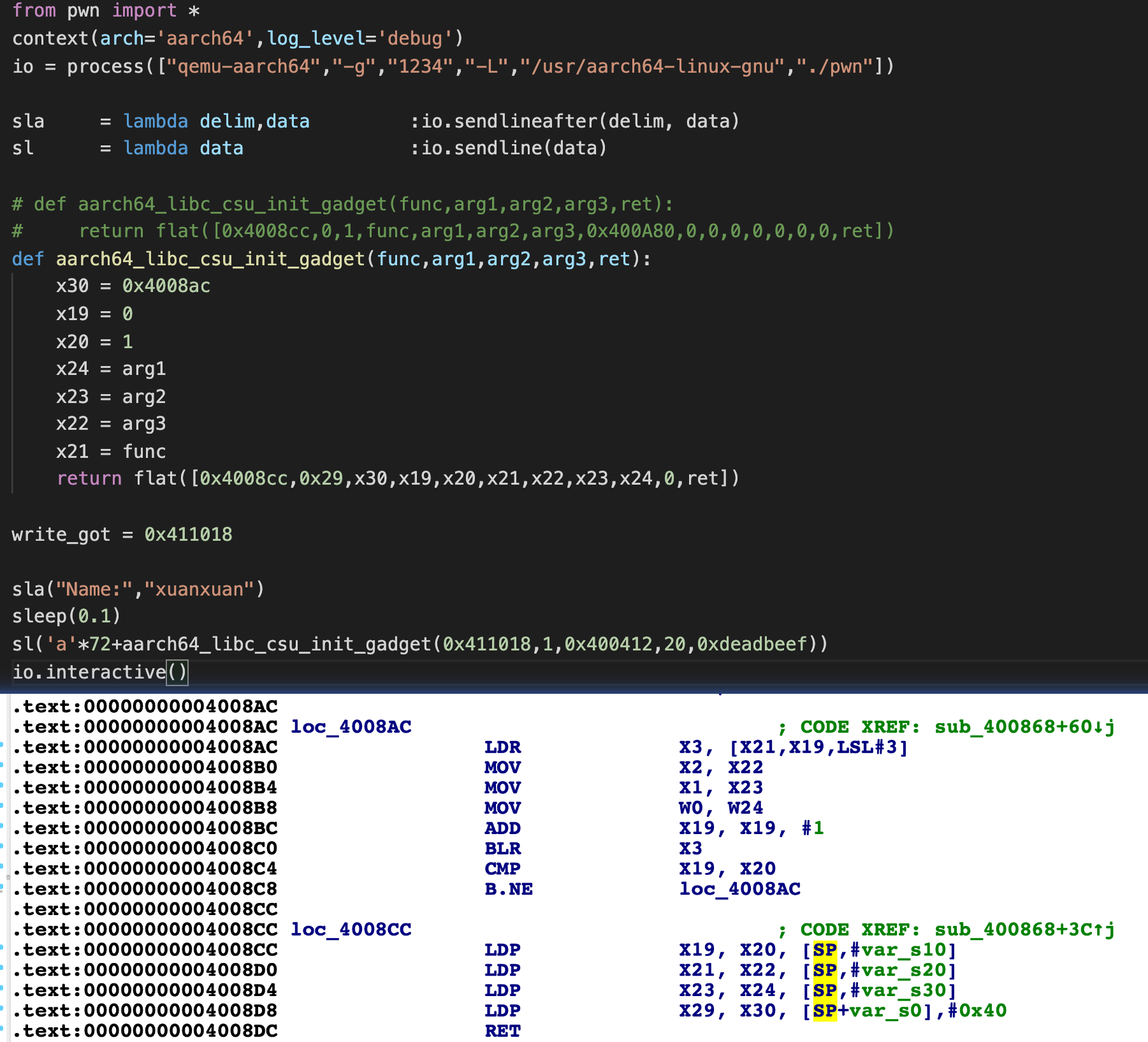## 历史考古

``````6.1 Why is this gadget here?
First of all, the complexity of the glibc is so high that it is very hard to find the ultimate
reason for some design decisions. Some design choices were motivated by other architecture
restrictions which are not applicable to ours. In other cases, the fear to break others
code or to cause baroque backward compatibility issues makes the developers to follow
the solid premise that "if it ain’t broke, don’t fix it".
``````## 我的理解

CSU 的含义是 “C Start Up” : What does CSU in glibc stand for?

``````void __libc_csu_init (int argc, char **argv, char **envp)
{
_init ();
const size_t size = __init_array_end - __init_array_start;
for (size_t i = 0; i < size; i++)
(*__init_array_start [i]) (argc, argv, envp);
}
``````

1. 函数的三个参数参数会原封不动的透传到下一个通过函数指针调用的函数中
2. 并且在透传之前还有其他的函数调用

1. 存在可以直接控制流劫持的寄存器
2. 参数寄存器由于其他函数调用不得不备份到其他寄存器
3. 当透传函数被调用时又必然要从其他寄存器中恢复参数
4. 若其他寄存器是函数调用上下文不易失的，则被调用函数需完成寄存器的备份与恢复（如同mips的s系列寄存器）
5. 大量寄存器的备份与恢复就是靠栈，这与指令集无关，或者说大家都是这么干的

1. 先从栈中恢复其他寄存器
2. 然后从其他寄存器恢复参数与控制流寄存器

## 编码测试

``````➜  uname -a
Linux ubuntu 4.15.0-162-generic #170-Ubuntu
➜  gcc -v
7.5.0-3ubuntu1~18.04
``````

``````int(*p)(int,int,int);

int c(int x,int y,int z){
return x+y+z;
}

int b(){
return 0;
}

int a(int x,int y,int z){
b();
p(x,y,z);
return 0;
}

int main(){
p = c;
a(1,2,3);
}
``````

``````➜  gcc test.c -o  test
``````

IDA观察，结果是不用费力倒腾寄存器了，直接从栈上出来了，但栈是由rbp寻址的，不能直接利用：

``````; Attributes: bp-based frame

public a
a proc near

var_C= dword ptr -0Ch
var_8= dword ptr -8
var_4= dword ptr -4

; __unwind {
push    rbp
mov     rbp, rsp
sub     rsp, 10h
mov     [rbp+var_4], edi
mov     [rbp+var_8], esi
mov     [rbp+var_C], edx
mov     eax, 0
call    b
mov     rax, cs:p
mov     edx, [rbp+var_C]
mov     esi, [rbp+var_8]
mov     ecx, [rbp+var_4]
mov     edi, ecx
call    rax ; p
mov     eax, 0
leave
retn
; } // starts at 621
a endp
``````

``````int(*p)(int,int,int);

int c(int x,int y,int z){
return x+y+z;
}

int b(){
return 0;
}

int a(int x,int y,int z){
b();
for(int i=0;i<10;i++){
p(x,y,z);
}
return 0;
}

int main(){
p = c;
int x,y,z = 1;
a(x,y,z);
}
``````

``````➜  gcc test.c -O2 -o test
``````

``````.text:0000000000000660 loc_660:                                ; CODE XREF: a+2F↓j
.text:0000000000000660                 mov     edx, r12d
.text:0000000000000663                 mov     esi, r13d
.text:0000000000000666                 mov     edi, r14d
.text:0000000000000669                 call    qword ptr [rbp+0]
.text:000000000000066C                 sub     ebx, 1
.text:000000000000066F                 jnz     short loc_660
.text:0000000000000671                 pop     rbx
.text:0000000000000672                 xor     eax, eax
.text:0000000000000674                 pop     rbp
.text:0000000000000675                 pop     r12
.text:0000000000000677                 pop     r13
.text:0000000000000679                 pop     r14
.text:000000000000067B                 retn
``````

## 修复方案

1. 函数的三个参数参数会原封不动的透传到下一个通过函数指针调用的函数中
2. 并且在透传之前还有其他的函数调用

1. __init函数必须在
2. 调__libc_csu_init时参数必须传好
3. 未来调函数指针时也必须要用这些参数

``````int(*p)(int,int,int);

int c(int x,int y,int z){
return x+y+z;
}

int b(){
return 0;
}

int xx,yy,zz;

int a(int x,int y,int z){
xx = x;
yy = y;
zz = z;
b();
for(int i=0;i<10;i++){
p(xx,yy,zz);
}
return 0;
}

int main(){
p = c;
int x,y,z = 1;
a(x,y,z);
}
``````

``````.text:0000000000000650                 push    rbp
.text:0000000000000651                 push    rbx
.text:0000000000000652                 lea     rbp, p
.text:0000000000000659                 mov     ebx, 0Ah
.text:000000000000065E                 sub     rsp, 8
.text:0000000000000662                 mov     cs:xx, edi
.text:0000000000000668                 mov     cs:yy, esi
.text:000000000000066E                 mov     cs:zz, edx
.text:0000000000000674                 jmp     short loc_692
.text:0000000000000674 ; ----------------------------------------------------------
.text:0000000000000676                 align 20h
.text:0000000000000680
.text:0000000000000680 loc_680:                                ; CODE XREF: a+48↓j
.text:0000000000000680                 mov     edx, cs:zz
.text:0000000000000686                 mov     esi, cs:yy
.text:000000000000068C                 mov     edi, cs:xx
.text:0000000000000692
.text:0000000000000692 loc_692:                                ; CODE XREF: a+24↑j
.text:0000000000000692                 call    qword ptr [rbp+0]
.text:0000000000000695                 sub     ebx, 1
.text:0000000000000698                 jnz     short loc_680
.text:000000000000069E                 xor     eax, eax
.text:00000000000006A0                 pop     rbx
.text:00000000000006A1                 pop     rbp
.text:00000000000006A2                 retn
``````

## 版本演变

``````static void
call_init (int argc, char **argv, char **envp)
{
/* For static executables, preinit happens right before init.  */
{
const size_t size = __preinit_array_end - __preinit_array_start;
size_t i;
for (i = 0; i < size; i++)
(*__preinit_array_start [i]) (argc, argv, envp);
}

# if ELF_INITFINI
_init ();
# endif

const size_t size = __init_array_end - __init_array_start;
for (size_t i = 0; i < size; i++)
(*__init_array_start [i]) (argc, argv, envp);
}
``````